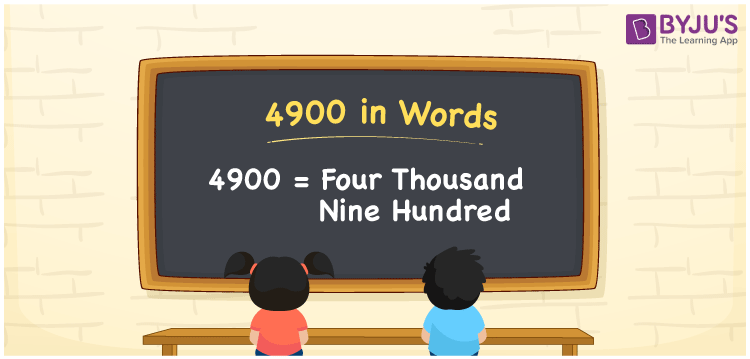# 4900 in Words

In English, the number 4900 is written as Four Thousand Nine Hundred. If you bought a jacket worth Rs. 4900, you can say, “I have bought a jacket worth Rupees Four Thousand Nine Hundred”. The number 4900 is a cardinal number. In this article, students are provided to learn how to read and write numbers in English in a comprehensive manner.

 4900 in words Four Thousand Nine Hundred Four Thousand Nine Hundred in numerical form 4900

## 4900 in English Words

Generally, numbers in words are expressed using the English alphabet. Thus, we can read 4900 in English as Four Thousand Nine Hundred.## How to Write 4900 in Words?

Let us have a look at the place value chart mentioned below for the conversion of the number 4900 to words.

 Thousands Hundreds Tens Ones 4 9 0 0

Thus, the expanded form can be written as:

4 x Thousand + 9 x Hundred + 0 × Ten + 0 × One

= 4 x 1000 + 9 x 100 + 0 x 10 + 0 x 1

= 4000 + 900 + 0 + 0

= 4000 + 900

= 4900

= Four Thousand Nine Hundred

Thus, 4900 in words is written as Four Thousand Nine Hundred

Interesting way of writing 4900 in words

4 = Four

49 = Forty-Nine

490 = Four Hundred and Ninety

4900 = Four Thousand Nine Hundred

Hence, the word form of the number 4900 is Four Thousand Nine Hundred

4900 is a natural number that precedes 4901 and succeeds 4899

• 4900 in words – Four Thousand Nine Hundred
• Is 4900 an odd number? – No
• Is 4900 an even number? – Yes
• Is 4900 a perfect square number? – Yes
• Is 4900 a perfect cube number? – No
• Is 4900 a prime number? – No
• Is 4900 a composite number? – Yes

## Frequently Asked Questions on 4900 in Words

Q1

### How do you write 4900 in words?

4900 in words is written as Four Thousand Nine Hundred.
Q2

### Write the value of 3600 + 1300 in words.

3600 + 1300 = 4900 Thus, the value of 3600 + 1300 in words is written as Four Thousand Nine Hundred.
Q3

### Is 4900 a composite number?

Yes, 4900 is a composite number.